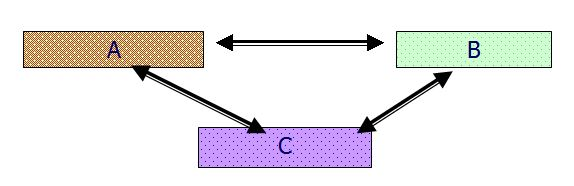There are four laws of thermodynamics as follows:
Zeroth law of thermodynamics:

When two systems are each in thermal equilibrium with a third system, then the two systems are also in thermal equilibrium with one another.First law of Thermodynamics:
• The heat and mechanical work are mutually convertible.
∂Q =∂W
• The energy can neither be created nor destroyed through it can be transformed from one form to another.
∂Q-∂W=dE

Second law of thermodynamics:
• Kelvin-Plank Statement: It is impossible to construct an engine working on a cyclic process, whose sole purpose is to convert heat energy from a single thermal reservoir into an equivalent amount of work.
• Clausius Statement: It is impossible for a self acting machine working on a cyclic process, to transfer heat from a body at a lower temperature to a body at a higher temperature without the aid of an external agency.
Third law of thermodynamics:

The entropy of any pure substance is thermodynamics equilibrium approaches zero as the temperature approaches zero.